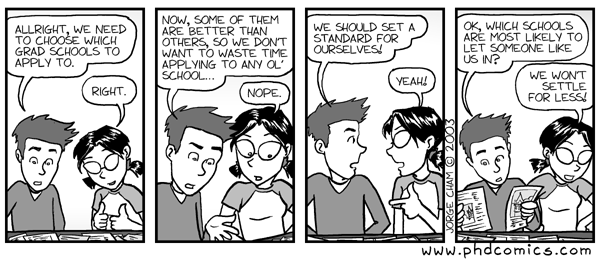# CSCE 782: Problem Set 3

## Due: Monday, 20 November 2006 @2:30pm

I could not make up my mind which problem set to assign so I am giving a choice to do any one of the following exercises.

## Soduko

The game of Soduko is a constraint satisfiaction problem. If you think about it, you quickly realize that each location in the grid is a variable whose domain is the range 1 to 9 and the constraints are given by the puzzle: no repetition of numbers on every row, column, or square. The numbers they give you are really part of the solution.

For this problem set you will write a program that solves (generates?) Soduko puzzles by generating all the numbers in the grid. Your program should also work if the user types in numbers in the orginally empty grid. That is, your program will solve the Soduko puzzle. Feel free to use our code for solving the graph coloring problem; I recommend using the distributed breakout algorithm as it does not need to generate no-goods (which are tricky to implement correctly). Note that I do not know how long it will take to solve a puzzle. I assume that the case with no numbers on the puzzle should be easy.

## Dynamo

In class I showed you how the Dynamo system generates pretty pictures which show the evolution of populations using the replicator dynamics. For this problem set you will implement one such demonstration. You can choose from any one of the ones he presents: the triangle (symmetric games with 3 actions), the square (general games with 2 actions and 2 populations), or the tetrahedron and cube (but you will need to use the 3D netlogo). Remember that in these visualizations every point represents a population.

Tip: You can set the patches to be the size of 1 pixel (1 by 1) and ask them to color themselves appropiately. The lines could be done dynamically by letting the user drop a turtle in any patch (population) and the having the turtle move around following the evolution of that particular population.

## Fictitious Dynamo

Similar to the previous problem, write a program that lets the user enter a game and then shows how two agents playing that game using fictitious play would change their strategies. We are looking for a visualization of the strategies' history.

## Mailmen Problem

Implement the mailmen problem (old non-working version is on website) where a number of mailmen is randomly placed in the grid with a number of letters to deliver. Each letter has a specific location that it needs to go to. The mailmen must return to the spot where they started at. The cost (negative utility) to each mailman is the length of its route (yes, this requires solving a traveling salesman problem but you can do that with a simple depth-first search, assuming the number of letters remains small, which we do).

You will then then calculate the various solutions to this bargaining problem, namely: the egaletarian social welfare, the utilitarian, the nash bargaining deal. This calculation will likely be centralized and will likely take some time. Finally, each solution should be graphically represented in the world.

### Submission Instructions

As will all the problem sets, you will hand them in using our department's dropbox.José M. Vidal